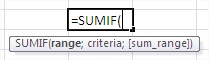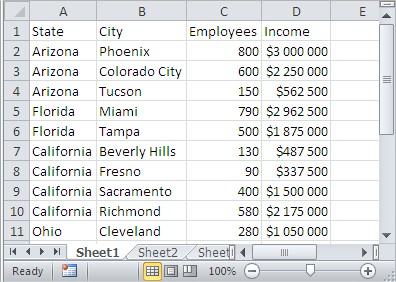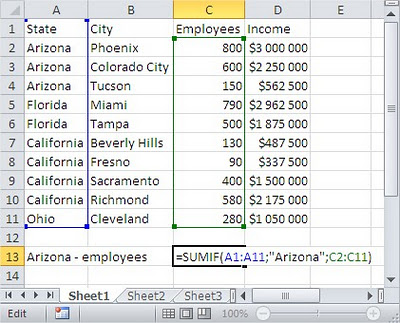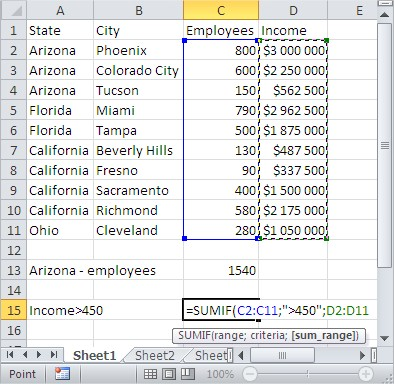#### How to Use Sumif Function in Excel

SUMIF is a built-in Excel function that sums the values in a range of cells based on a given criteria. It is a very versatile function that can be used in a variety of ways.

## What is SUMIF in Excel?

SUMIF stands for “Sum If”. It is a function that takes three arguments:

• The range of cells you want to sum
• The criteria for the sum
• The optional range of cells for the criteria

For example, if you have a range of cells that contains sales figures, and you want to sum the sales for all customers in California, you would use the following formula:
=SUMIF(A2:A100, “California”, B2:B100)

This formula would sum the values in the range B2:B100 where the corresponding cells in the range A2:A100 equal “California”.

## How SUMIF works

SUMIF works by first searching for the criteria you specify in the first argument. If it finds a match, it sums the values in the range you specify. If it doesn’t find a match, it returns 0.

The optional third argument can be used to specify a different range for the criteria. This can be useful if the criteria is not in the same range as the values you want to sum.

## Syntax of SumIf function

The syntax of the function: =SUMIF(range,criteria,[sum_range])Mandatory argument range is the range of cells you want to estimate the function. This is the range of cells, where the user desire to apply a criteria against.

The criteria argument is used to determine the criteria against which the function will be summarized cells. It is possible to use the criterion in terms of numbers, Boolean expression, or text.

Sum_range use the optional argument, if we want to identify the specific cells that will be summed, where the corresponding cell in the field meet the criteria. If omitted, the function suma_range will add up all cells in the range.

This is an optional argument, because it is the cells that you’d might like to sum. However, if this part of argument is omitted, then the function would make use of range as its sum_range.

## Sumif function examples

Usage of SUMIF explains the following example.To calculate the sum of people employed in Arizona, the cell type in the formula:

=SUMIF(A2:A11,”Arizona”,C2:C11)As you can see, in this case the range of cells, where we check the criterion (A2: A11) is different from that which we value sum (C2: C11). Please also note that the criterion for entering text, enclose it in quotation marks. In the case of the criterion being the number of quotes can be omitted.

To calculate the total income in units employing more than 450 people, we use the formula:

=SUMIF(C2:C11,”>450″,D2:D11)Like the search text, logical expressions apprehend in quotation marks.

## Tips

You can use text, numbers, or dates as criteria in a SUMIF formula.
You can use wildcards in a SUMIF formula to match multiple values. For example, the formula =SUMIF(A2:A100, “*West*”, B2:B100) would sum the sales for all customers whose names contain the word “West”.
You can use the SUMIFS function to sum the values in a range of cells based on multiple criteria.